## Exploring Matlab via the T1 and T2 decay curves

Let's explore the decay curves for T1 and T2 MRI signals. Along the way, we'll learn about some basic matlab commands. We'll try to reproduce this figure from the Principles of fMRI Slides (Module 5):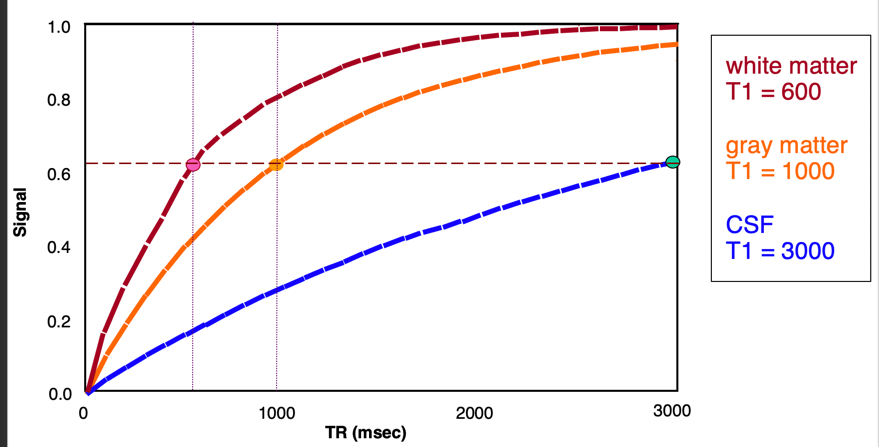### The editor

First, the editor. Type:
edit
This opens the Matlab editor, in which you can edit scripts and functions.
This lab is a "live script", which combines text and editable code. It's very popular now to use this kind of script to create a "notebook", a living document with code you can run and results.
The most popular of these is Jupyter notebooks for Python, which also supports notebooks in other languages, including Matlab. Here, we'll use Matlab's built-in live editor.
You can open this script in the editor.

### T1 and T2 curves: Exponential growth/decay

Now, let's explore the T1 and T2 decay curves.
These take the form of exponential growth curves. The curve is increased or decreased by some proportion of an original quantity at each time step. These thus take the form: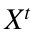, where t is time. If the exponent is positive, the curve will have an increasing slope over time (e.g., like bacteria doubling, or money doubling with compound interest). But if it's negative, the curve will have a decreasing slope over time, and converge on any asymptote. The T1 and T2 decay curves are both exponential curves with negative exponents.
These curves are modeled (described) using the number e as the constant. e is defined so that the natural logarithm of e is 1, one of the "universal constants" in nature. It's approximately 2.718. In Matlab, exp(x) is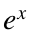, so exp(1) = e.
One point from the slides is that the T1 relaxation time is defined as the time where the signal recovers 0.63 of the way to full recovery. Likewise, the T2 time is the time it takes to decay to 0.37 (1 - 0.63). One question from the slides is, "where does the contstant 0.63 come from?" This falls out of using e as the base for the equation. Try this:
1/exp(1)
ans = 0.3679
What do you get?

### T1 equation and plot

Now let's build up the equations for the T1 and T2 curves. They are also scaled by a constant, Mo. So the form is: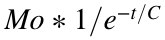, where Mo is a constant that relates to the magnetization strength, and C is a constant related to decay...either T1 or T2.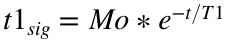We'll leave off the constant Mo for now, and define this equation in Matlab. We'll use something kind of advanced here for this example, but don't worry if it doesn't make perfect sense now. We'll define a function of time, f(t), with the T1 equation. We'll use the T1 constant for gray matter, 1000 msec.
Note: use % for comments, which is very very helpful in code!
T1 = 1000; % T1 time for gray matter
t1relaxation = @(t) 1 - 1./exp(t./T1); % define function of t
t1relaxation is the name of a variable -- it stores the "handle" to our new function. (This is called an "anonymous function" in Matlab; I've defined it from the command line). I can pass in any value of t, and get the result back out. Let's try it:
t1relaxation(500)
ans = 0.3935
If i run this for 1000 (the gray-matter constant), what value will I get?
t1relaxation(1000)
ans = 0.6321
I get 0.63! So i've recovered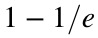of the signal.
Now, we'll create a whole vector of times, and use it to make a plot. I use the brackets [ ] to define the vector, and the : to specify a range:
t = 1:2000; % a vector of times in msec
whos t
Name Size Bytes Class Attributes t 1x2000 16000 double
Whos t tells us that t is a vector with 1 row and 2000 columns.
I can look at the first of these values like this, using ( ) to specify which values of t we want:
t(1:10) % first 10 values of t
ans = 1×10
1 2 3 4 5 6 7 8 9 10
Now let's make a figure and plot all these values. We'll put some labels on it, too:
figure; % Create a new figure
hold on; % Leave previous graphics on when adding new ones
% plot(x-axis values, y-axis values)
plot(t, t1relaxation(t))
xlabel('t(msec)')
ylabel('Signal');
title('T1 relaxation')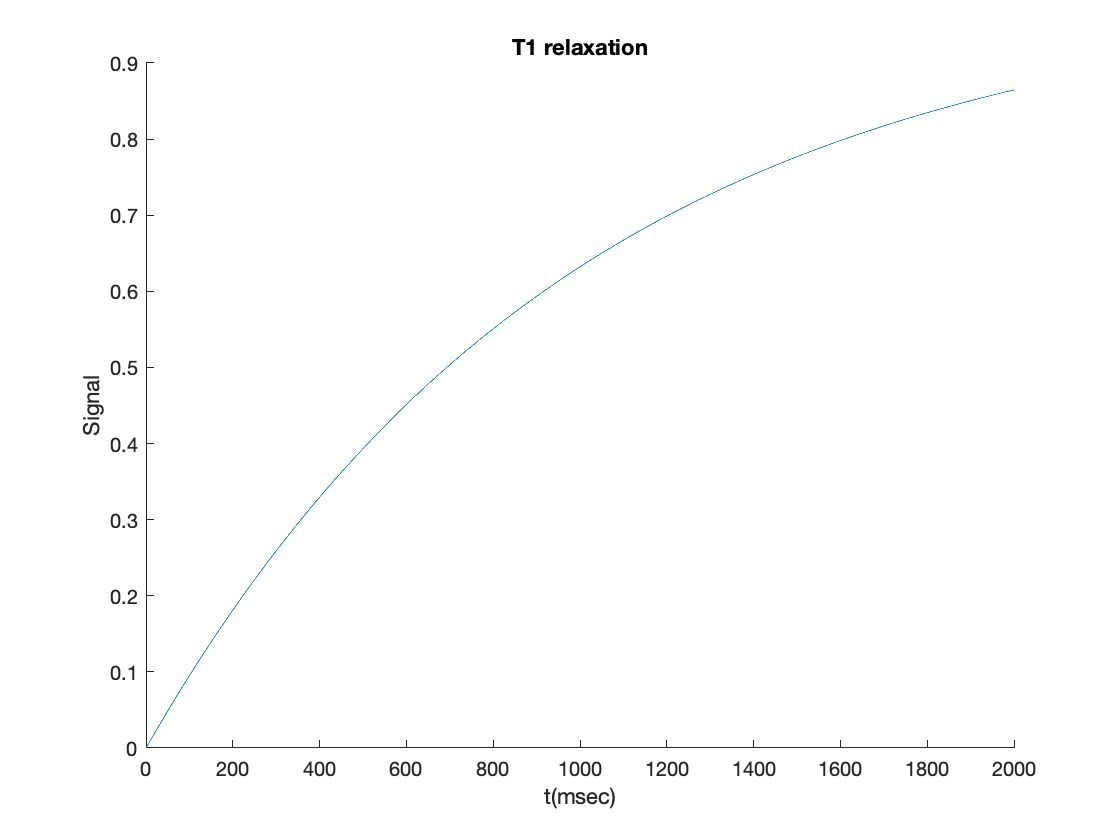Matlab uses a "handle" system for graphics, where you can create variables that are pointers to graphical objects (lines, surfaces, text labels, and other objects). You can change the properties of these objects, and it will change the look of the graph. Let's clear the current figure and create a new plot, with lines that match the figure at the start of the exercise a bit better:
cla % clear current axis
plot(t, t1relaxation(t), 'Color', [1 .3 .7], 'LineStyle','--', 'LineWidth', 3);
xlabel('t(msec)')
ylabel('Signal');
title('T1 relaxation')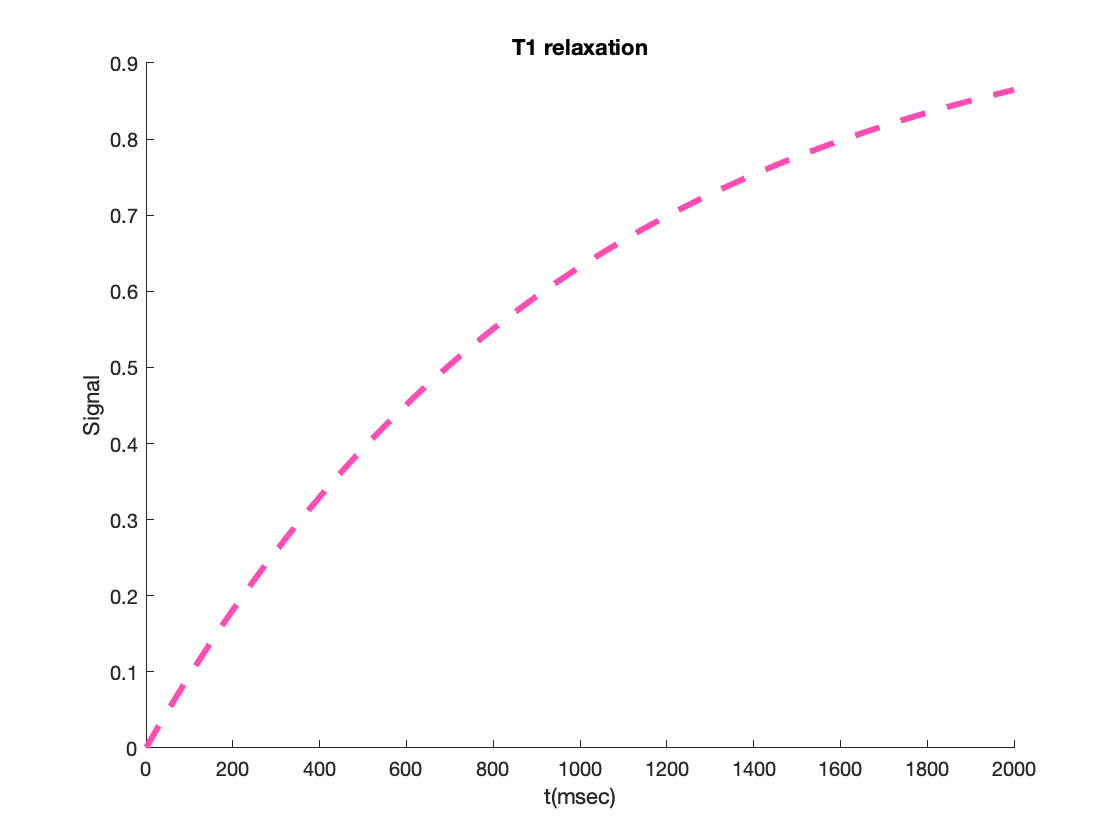A note about colors: Matlab uses a 3-element vector with [Red Green Blue] values, always between 0 and 1. They combine as do the colors of light. [1 1 1] is white. [0 0 0] is black. Try changing the color to something interesting!

### The final plot

Now we'll add some other lines, for different tissue types, and a legend.
But first, we have to redefine the anonymous function t1relaxation to take TWO inputs. The inputs are defined at the start of the command, @( ). The @ says this is going to be a function, and the ( ) tell it what inputs to expect. Before, we "baked in" the gray matter constant (1000) because it wasn't specified as a free input variable. The only input argument was t. Now, we'll pass in t and a tissue-specific T1 constant:
t1relaxation = @(t, T1) 1 - 1./exp(t./T1); % define function of t and T1 constant
% Next, calculate the signal values for each tissue type and save them in
% variables:
t1gray = t1relaxation(t, 1000);
t1white = t1relaxation(t, 600);
t1csf = t1relaxation(t, 3000);
Now, we're finally ready to plot all these together:
figure;
hold on;
plot(t, t1gray, 'Color', [1 .5 0], 'LineStyle','--', 'LineWidth', 3);
plot(t, t1white, 'Color', [1 0 0], 'LineStyle','--', 'LineWidth', 3);
plot(t, t1csf, 'Color', [0 0 1], 'LineStyle','--', 'LineWidth', 3);
xlabel('t(msec)')
ylabel('Signal');
title('T1 relaxation')
legend({'Gray' 'White' 'CSF'}) % legend expects a cell input with names (strings). Use { } for cells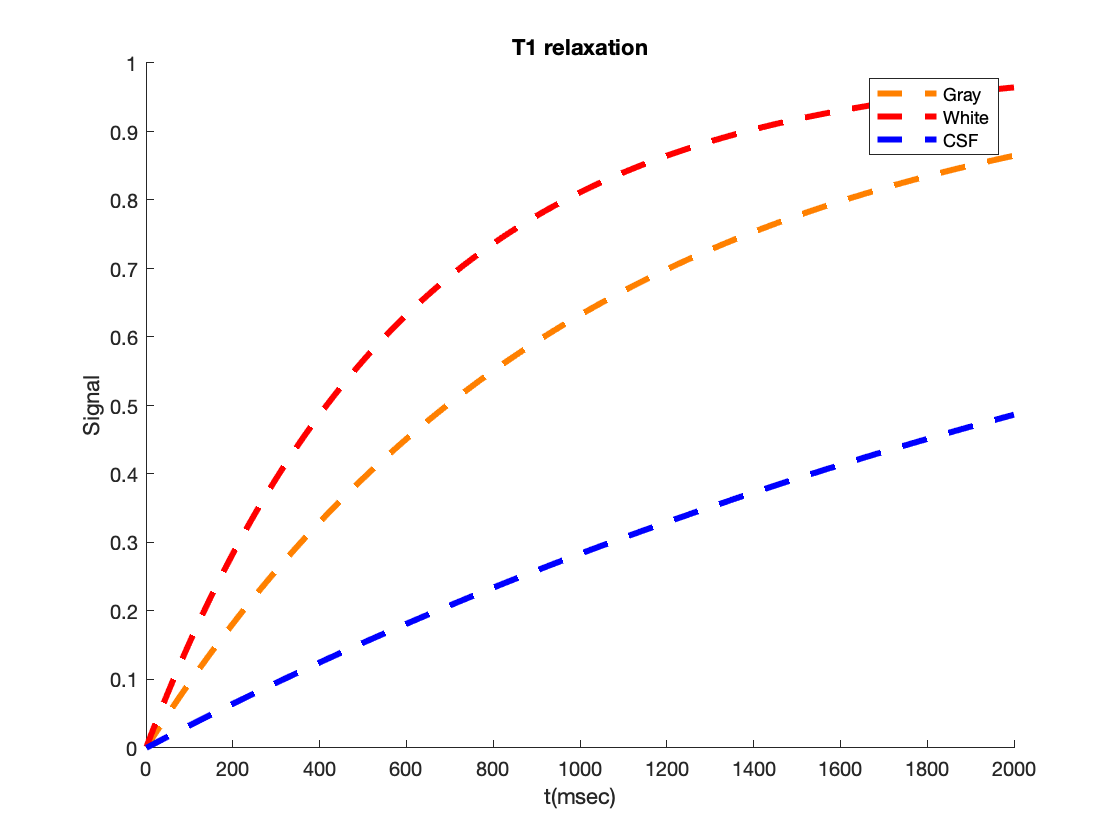For a finishing touch, we'll draw some vertical lines at the T1 constant, and a horizontal line where it crosses the 0.63 line. You can do all this in native Matlab code (no toolboxes), but just for fun, and to check if you have the CANlab tools installed, we'll take advantage of one of the CANlab tools: A function called plot_vertical_line.
In addition, we'll illustrate some more properties of handle graphics. When we make the plot, we'll pass out the handle to the line in a variable called han. We can change its properties after making the plot, and it will change the plot!
han = plot_vertical_line(1000); % T1 for gray matter
set(han, 'Linestyle', ':', 'Color', [1 .5 0]);
han2 = plot_vertical_line(600); % T1 for white matter
set(han2, 'Linestyle', ':', 'Color', [1 0 0]);
% Now horizontal line:
han3 = plot(t, t1relaxation(1000, 1000) * ones(size(t)), 'LineStyle', ':');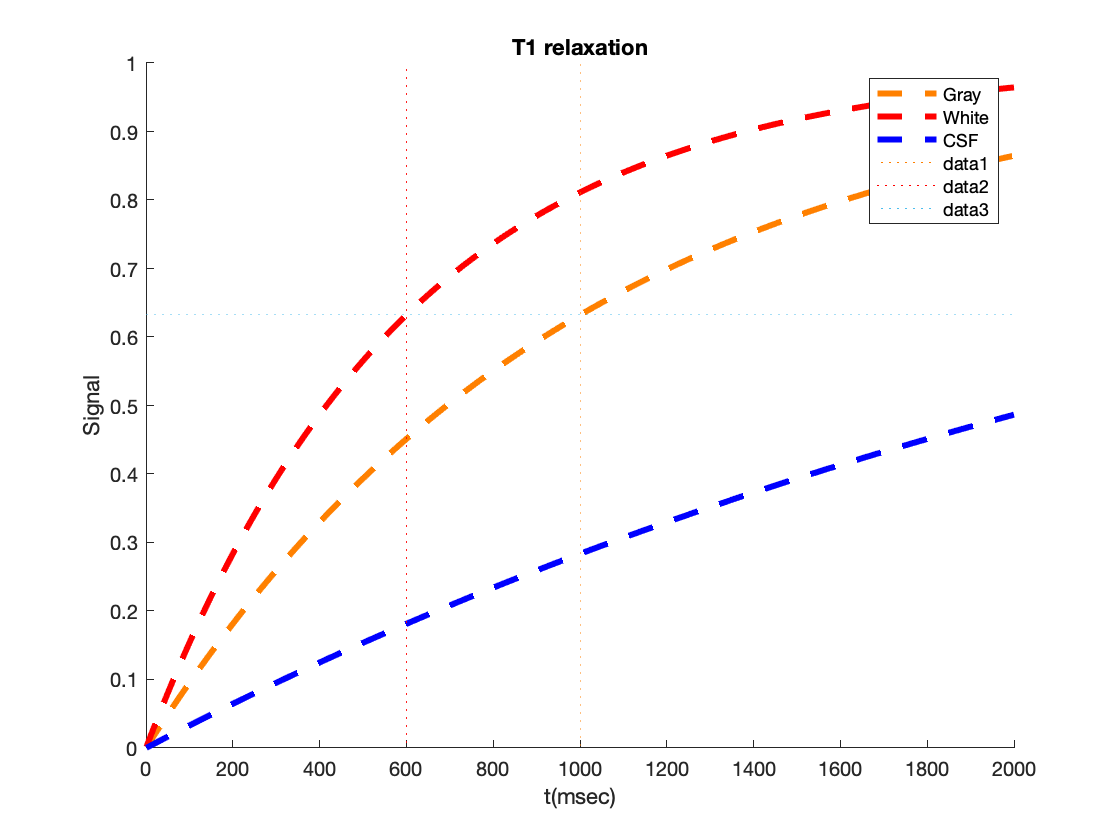Voila! We did it.
NOTE: if you get Unrecognized function or variable 'plot_vertical_line' , you need the CANlab Core tools on your Matlab path.
Let's break this down a bit.
The last plot( ) command involved two elements: First, finding the y-axis value for the horizontal line, the signal value at the T1 constant (0.63), but precisely. The code t1relaxation(1000, 1000) does this.
Second, we've mulitplied this by a vector of ones, e.g., [1 1 1 1 1], with the same number of ones as in the vector t. The format of plot is plot(x, y), so in this case, plot(x=t, y=0.63). Because we have 2000 elements for t, we need 2000 elements for y as well. But the y value is the same for every x (that defines a horizontal line). So we create the y vector 0.63 * [1 1 1 1 1....]. This is done with ones(size(t)). It invokes a function, size( ), which gets the number of elements in t. Try size(t) and you'll get [1 2000] back out. This is the number of rows and columns in t. Now, we'll pass that directly into the function ones( ), which creates a matrix of ones, and takes an input argument with the number of rows and columns you want for the matrix. Passing in [1 2000], we get a row vector of ones the same size as t. Then we can plot each element of t against each element of this new vector, which plots a horizontal line.
This illustrates a few basic Matlab functions, including size(), ones(), and the concept of passing results from one function directly into another via chained commands like ones(size(t)).
Finally, a bit more about function handles.
In the code above, han is a handle to a line with many properties. I can find out what they are with get( ), and change them with set( ). e.g.,
get(han)
AlignVertexCenters: off Annotation: [1×1 matlab.graphics.eventdata.Annotation] BeingDeleted: off BusyAction: 'queue' ButtonDownFcn: '' Children: [0×0 GraphicsPlaceholder] Clipping: on Color: [1 0.5000 0] ColorMode: 'manual' ContextMenu: [0×0 GraphicsPlaceholder] CreateFcn: '' DataTipTemplate: [1×1 matlab.graphics.datatip.DataTipTemplate] DeleteFcn: '' DisplayName: 'data1' HandleVisibility: 'on' HitTest: on Interruptible: on LineJoin: 'round' LineStyle: ':' LineStyleMode: 'manual' LineWidth: 0.5000 Marker: 'none' MarkerEdgeColor: 'auto' MarkerFaceColor: 'none' MarkerIndices: [1 2] MarkerMode: 'auto' MarkerSize: 6 Parent: [1×1 Axes] PickableParts: 'visible' Selected: off SelectionHighlight: on SeriesIndex: 4 Tag: '' Type: 'line' UserData: [] Visible: on XData: [1000 1000] XDataMode: 'manual' XDataSource: '' YData: [0 1] YDataSource: '' ZData: [1×0 double] ZDataSource: ''
set(han, 'Color', [0 .7 1], 'LineWidth', 4)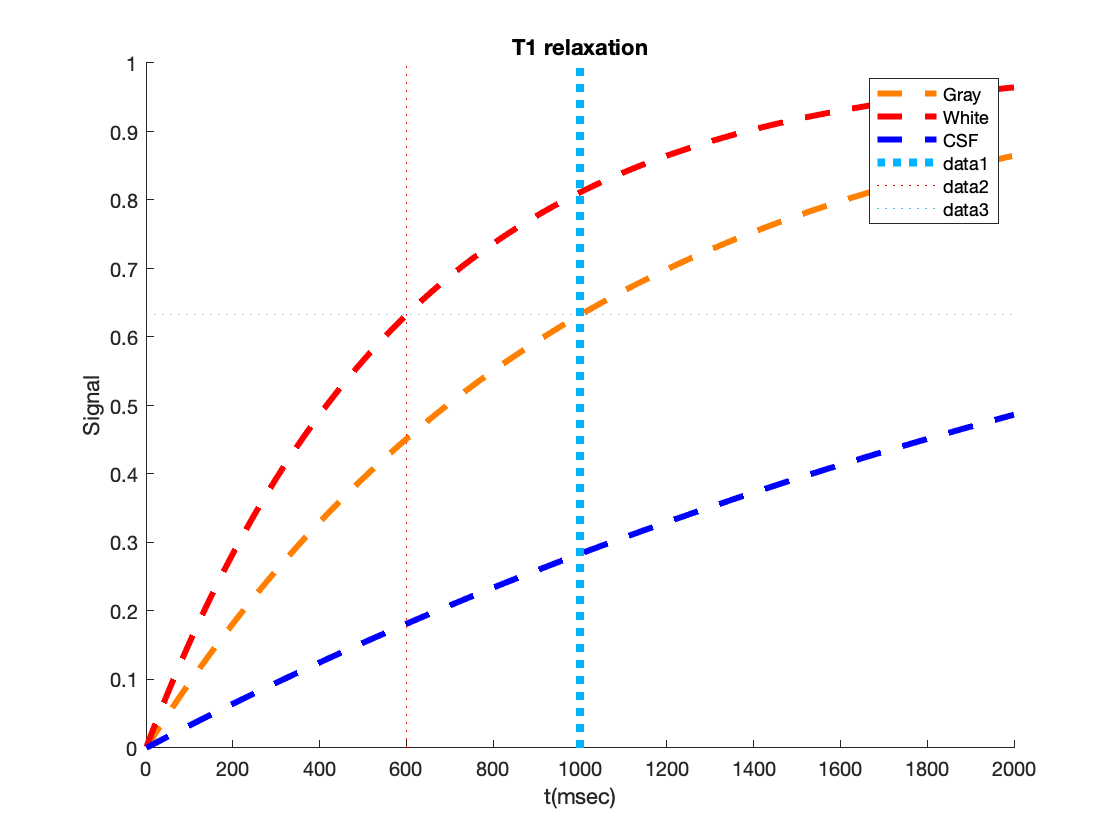### Matlab help

Finally, if you want more details on how these work, try typing help ones, help get, help set, etc. Let's try it:
help size
SIZE Size of array. D = SIZE(X), for M-by-N matrix X, returns the two-element row vector D = [M,N] containing the number of rows and columns in the matrix. For N-D arrays, SIZE(X) returns a 1-by-N vector of dimension lengths. Trailing singleton dimensions are ignored. [M,N] = SIZE(X) for matrix X, returns the number of rows and columns in X as separate output variables. [M1,M2,M3,...,MN] = SIZE(X) for N>1 returns the sizes of the first N dimensions of the array X. If the number of output arguments N does not equal NDIMS(X), then for: N > NDIMS(X), SIZE returns ones in the "extra" variables, i.e., outputs NDIMS(X)+1 through N. N < NDIMS(X), MN contains the product of the sizes of dimensions N through NDIMS(X). M = SIZE(X,DIM) returns the lengths of the specified dimensions in a row vector. DIM can be a scalar or vector of dimensions. For example, SIZE(X,1) returns the number of rows of X and SIZE(X,[1 2]) returns a row vector containing the number of rows and columns. M = SIZE(X,DIM1,DIM2,...,DIMN) returns the lengths of the dimensions DIM1,...,DIMN as a row vector. [M1,M2,...,MN] = SIZE(X,DIM) OR [M1,M2,...,MN] = SIZE(X,DIM1,...,DIMN) returns the lengths of the specified dimensions as separate outputs. The number of outputs must equal the number of dimensions provided. When SIZE is applied to a Java array, the number of rows returned is the length of the Java array and the number of columns is always 1. When SIZE is applied to a Java array of arrays, the result describes only the top level array in the array of arrays. Example: If X = rand(2,3,4); then d = size(X) returns d = [2 3 4] [m1,m2,m3,m4] = size(X) returns m1 = 2, m2 = 3, m3 = 4, m4 = 1 [m,n] = size(X) returns m = 2, n = 12 m2 = size(X,2) returns m2 = 3 See also LENGTH, NDIMS, NUMEL.
That's all for now!
As a bonus exercise, you might try plotting the T2 curve based on the lecture slides! (Nearly the same equation...)

### Debugger

Matlab's debugger is super useful! Debug mode commands start with db, like dbstack.
If you see the K>> prompt, you're in the debugger. You're "inside" a function, and can see the variables in the function workspace.
If you're stuck in debugger and want to get out: dbquit (may have to repeat this)
To turn it off: dbclear all
To turn it on: dbstop if error
This is great if you need to debug a function.